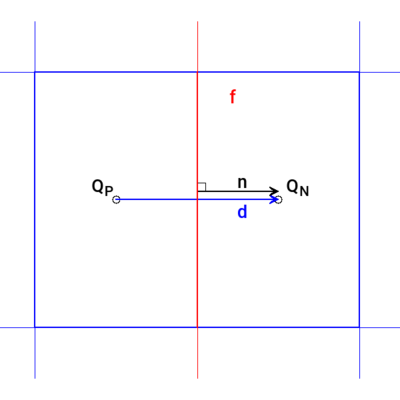The vector $$\vec{d}$$ joining two cell centres is parallel to the normal direction of the inter-connecting face.

$\snGrad Q = \frac{Q_P - Q_N}{| \vec{d} |}$## Properties🔗

• Calculated using a central difference scheme using the mesh delta coefficients
• Any non-orthogonality is ignored

## Usage🔗

The scheme is specified using:

snGradSchemes
{
default         none;